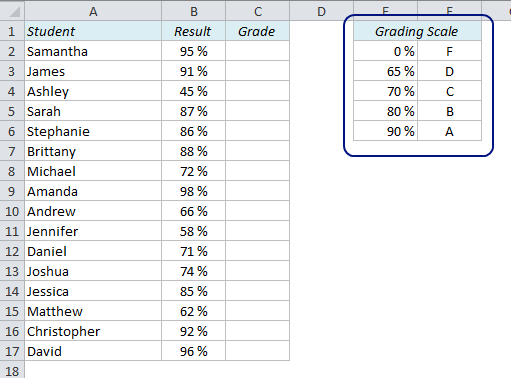How to Calculate Grades in Excel

How can we calculate the grades (A-F) in Excel if we have the test results as numbers? We know that a score of 90% or higher is an A, 80-89% is a B, 70-79% is a C, 65-69% is a D and less than 65% is an F.

The first thing we should do is to organize this information in a lookup table:How VLOOKUP can get you in trouble and how to solve it

An Extra Column Means Trouble

If you want to find a value in a table in Excel, a simple VLOOKUP function is usually a good and easy way to do it. But you have to be careful – if you insert a new column in your table, the function might not work anymore, and we have to find another approach. Here’s why: